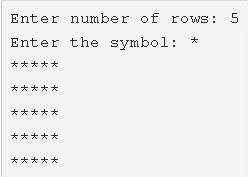# C Square Star Pattern Program – C Pattern Programs | C Programs

C program to print a square star pattern – In this article, we will detail in on the multiple means to print a square star pattern in C programming.

• Using For Loop
• Using While Loop
• Using Do-While Loop

As we all know, a square is an important figure in the field of geometry and life as well. A square is a two-dimensional quadrilateral figure which has equal sides and equal angles.

The angles of a square are 90 degrees. The opposite sides of a square are parallel in nature.As you can see, you need to enter the number of the rows first up.

Accordingly, your square will be printed. In this case, the number of rows entered is 5.

Then, go ahead with denoting the symbol with which you want to print the square with.

The ‘*’ symbol is chosen here to do the honours.

In the end, your square pattern will get printed according to the code entered.

Thus, the multiple means to do the same in C programming are as follows:

## Using For Loop

1. Read the row number and store that value into the variable n which is integer data type, read the entered character and store that character into the variable ‘ch’.

2) The outer for loop iterates through n rows with the structure  for(i=0;i<n;i++).

a) The inner for loop iterates through columns and prints n symbols in each row.

b) Cursor comes to next line after each row.

3) In given example n=5,

In each row, the 2nd for loop prints 5 stars. After all the iterations of outer for loop, we will get Square star pattern with 5 rows and 5 columns.

Output:

### Using While Loop

1) The while loop iterates through rows until the condition(i++<n) becomes false.

j=0

2) The inner while loop iterates through columns until the condition  (j++<n) becomes false.

a) prints symbol if the condition is true.

b) j value increased by 1.Repeates the inner loop iterates through columns until the condition becomes false.

3) Cursor comes to next line.

4) i value increased by 1.Repeates the outer loop until the condition becomes false.

Output:

#### Using Do-While Loop

1)For i=0 the outer do-while loop iterates through row

2) For j=0 the inner do-while loop iterates through the column.

a) prints the symbol.

b) j value increased by 1.

c) Check the condition(++j<n), if the condition is true then iterate once. Repeat the inner loop until the condition becomes false.

3) Cursor comes to next line.

4) a) i value increased by 1.

b) Check the condition (++i<n), if the condition is true then iterate once. Repeat the outer loop until the condition becomes false.

Output:

x

## C Program To Print Number Of Days In A Month | Java Tutoring

C program to input the month number and print the number of days in that ...